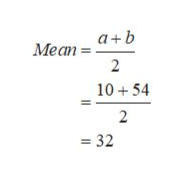A random number generator picks a number from 10 to 54 in a uniform manner. Round answers to 4 decimal places when possible.The mean of this distribution is The standard deviation is The probability that the number will be exactly 12 is P(x = 12) = The probability that the number will be between 19 and 49 is P(19 < x < 49) = The probability that the number will be larger than 33 is P(x > 33) = P(x > 21 | x < 37) = Find the 92nd percentile. Find the minimum for the lower quartile.

Question

A random number generator picks a number from 10 to 54 in a uniform manner. Round answers to 4 decimal places when possible.

The mean of this distribution is
The standard deviation is
The probability that the number will be exactly 12 is P(x = 12) =
The probability that the number will be between 19 and 49 is P(19 < x < 49) =
The probability that the number will be larger than 33 is P(x > 33) =
P(x > 21 | x < 37) =
Find the 92nd percentile.
Find the minimum for the lower quartile.

Step 1

Hello. Since your question has multiple sub-parts, we will solve first three sub-parts for you. If you want remaining sub-parts to be solved, then please resubmit the whole question and specify those sub-parts you want us to solve.

Consider a random variable X which follows uniform distribution with parameters a and b equals to 10 and 54 respectively.

The mean of the distribution is,help_outlineImage TranscriptioncloseМеaп 3 Meanab 1054 2 = 32 fullscreen
Step 2

The standard devi...

Want to see the full answer?

See Solution

Want to see this answer and more?

Our solutions are written by experts, many with advanced degrees, and available 24/7

See Solution
Tagged in

Advanced Topics in Statistics